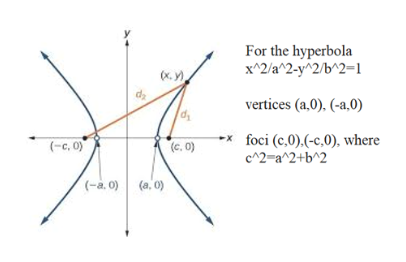# Find the vertices and locate the foci for the hyperbola whose equation is given.64x2 - 49y2 = 3,136

Question
20 views

Find the vertices and locate the foci for the hyperbola whose equation is given.

64x2 - 49y2 = 3,136

check_circle

Step 1

To identify the vertices and the foci of the given hyperbola

Step 2

Verices and foci for the sta...help_outlineImage TranscriptioncloseFor the hyperbola x^2/a^2-y^2/b^2=1 vertices (a,0), (-a,0) foci (c,0).(-c,0), where c^2-a^2+b^2 x (c. O) (c. 0) (-à. 0) (a, o) fullscreen

### Want to see the full answer?

See Solution

#### Want to see this answer and more?

Solutions are written by subject experts who are available 24/7. Questions are typically answered within 1 hour.*

See Solution
*Response times may vary by subject and question.
Tagged in

### Math